# Formula Expression

This uses a formula to create the necessary condition and this formula can refer to multiple cells.
You can pretty much use any formula you like as long as it evaluates to either True or False.
If the formula evaluates to True then the condition is satisfied and the formatting will be applied.
If the formula evaluates to False then the formatting is not applied.
In the first drop-down box select "Formula Is".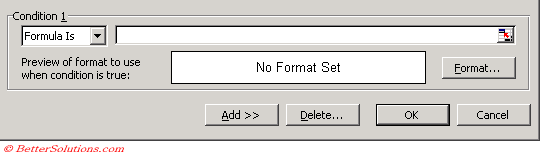You can either enter the formula directly or you can refer to a cell that contains the formula.

### Using a Formula

We are going to apply conditional formatting to the range of cells "B2:E5" and we are going to shade the cells which contain a number greater than 20.
Select the range of cells you want to apply the conditional formatting to, in this case cells "B2:E5".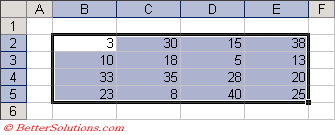Select (Format > Conditional Formatting) to display the Conditional Formatting dialog box.
Select "Formula Is" in the first drop-down box and enter the formula "B2<20".
If you want to refer to the active cell in the formula then you need to refer to the top left cell in the selected range, in this case cell "B2".
Click the Format button to apply your specific formatting, in this case we are just applying a grey background.
This is actually the (Format > Cells) dialog box but it only contains the Font, Border and Patterns tabs.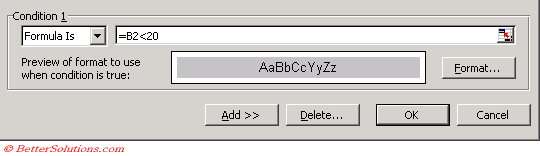Press OK to apply your conditional formatting.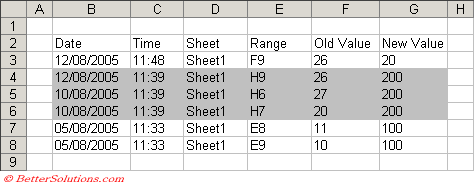### Using a Cell Reference

This almost identical to the above except that instead of entering the formula directly we can use a cell reference to the formula.
This approach can make it quick to change the condition which is used.
Select the range of cells you want to apply the conditional formatting to, in this case cells "B2:E5".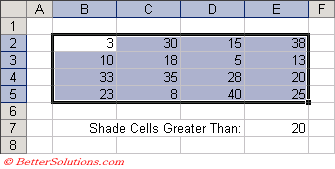Select (Format > Conditional Formatting) to display the Conditional Formatting dialog box and select "Formula Is" in the first drop-down box.
If you want to refer to the active cell in the formula then you need to refer to the top left cell in the selected range, in this case cell "B2".
Instead of entering a constant you can also use a cell reference, select the cell "E7".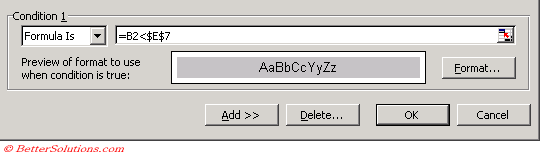Press OK to apply your conditional formatting.

=AND(
=OR(

### Important

If you enter a cell reference then it is assumed to be an absolute reference.
You can use any formula you like as long as returns a logical value (i.e. True of False).
You can only have a source reference to cells on the active sheet, although if you want to refer to a range on a different worksheet then you can use a named range.
If the formula contains a cell reference then the formula is referred to as "relative".